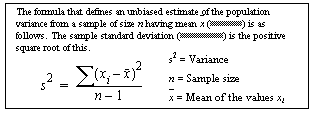# var_samp

Computes the statistical variance of a sample consisting of a numeric-expression, as a double, and returns the variance of a set of numbers. var and variance are aliases of var_samp, and use the same syntax.

## Syntax

`var_samp ( [ all | distinct] expression )`

## Parameters

• all – applies var_samp to all values. all is the default.
• distinct – eliminates duplicate values before var_samp is applied.
• expression – is any numeric datatype (float, real, or double) expression.

## Examples

• Example 1 – Lists the average and variance of the advances for each type of book in the pubs2 database:
```select type, avg(advance) as "avg", var_samp(advance)
as "variance" from titles where
total_sales > 2000 group by type order by type```

## Usage

var_samp returns a result of double-precision floating-point datatype. If applied to the empty set, the result is NULL.

Formula for Sample-Related Statistical Aggregate FunctionsFor general information about aggregate functions, see Aggregate Functions in Transact-SQL Users Guide.

## Standards

ANSI SQL – Compliance level: Transact-SQL extension.

## Permissions

Any user can execute var_samp.

Related reference
stddev_pop
stddev_samp
var_pop